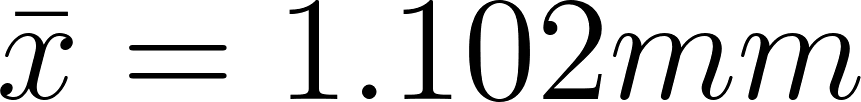×
Get Full Access to Statistics For Engineers And Scientists - 4 Edition - Chapter 6.1 - Problem 2e
Get Full Access to Statistics For Engineers And Scientists - 4 Edition - Chapter 6.1 - Problem 2e

×

# A simple random sample consists of 65 lengths of pianoISBN: 9780073401331 38

## Solution for problem 2E Chapter 6.1

Statistics for Engineers and Scientists | 4th Edition

• Textbook Solutions
• 2901 Step-by-step solutions solved by professors and subject experts
• Get 24/7 help from StudySoup virtual teaching assistantsStatistics for Engineers and Scientists | 4th Edition

4 5 1 370 Reviews
13
4
Problem 2E

Problem 2E

A simple random sample consists of 65 lengths of piano wire that were tested for the amount of extension under a load of 30 N. The average extension for the 65 lines was 1.102 mm and the standard deviation was 0.020 mm. Let µ represent the mean extension for all specimens of this type of piano wire.

a. Find the P-value for testing H0: ≤ 1.1 versus H1:µ > 1.1.

b. Either the mean extension for this type of wire is greater than 1.1 mm, or the sample is in the most extreme __________ % of its distribution.

Step-by-Step Solution:

Problem 2E

A simple random sample consists of 65 lengths of piano wire that were tested for the amount of extension under a load of 30 N. The average extension for the 65 lines was 1.102 mm and the standard deviation was 0.020 mm. Let µ represent the mean extension for all specimens of this type of piano wire.

a. Find the P-value for testing H0: ? 1.1 versus H1:µ > 1.1.

b. Either the mean extension for this type of wire is greater than 1.1 mm, or the sample is in the most extreme __________ % of its distribution.

Step by step solution:

Step 1:

Given,

Sample size,Mean,Standard deviation,Step 2 of 3

Step 3 of 3

##### ISBN: 9780073401331

Unlock Textbook Solution